# A solution to bandit convex optimization

Ronen Eldan and I have just uploaded to the arXiv our newest paper which finally proves that for online learning with bandit feedback, convex functions are not much harder than linear functions. The quest for this result started in 2004 with a paper by Robert Kleinberg, and one by Flaxman, Kalai, McMahan, and over the years it has become the flagship open problem in bandit theory (see for instance Hazan and Levy 2015 where they refer to the attainable regret for convex bandit as the most important open question in bandit learning).

Our result with Ronen however is far from the end of the story for this problem: currently our dependency on the dimension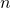is, which can certainly be improved, and even more importantly we do not yet know an algorithm which attains our regret bound! Indeed our proof follows the trail of my COLT’15 paper with Ofer Dekel, Tomer Koren and Yuval Peres where we suggest to attack the dual Bayesian problem (in the latter paper we solved this problem in dimension one). Thus finding an algorithm with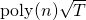for bandit convex optimization remains as open as before, but now at least we know that such an algorithm exist!!

Along the way of our new proof we solve an interesting property testing problem on convex functions, which we feel could have applications beyond convex bandits. The problem can be described as follows. Assume that you are given a convex and non-negative convex function. Now suppose that there is some unknown convex function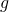defined on the same domain asand for which you would like to know ifis equal to. I also guarantee you that ifis not equal tothentakes a negative value somewhere on its domain (say even less than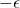). You can obtain information onthrough noisy queries, that is you can ask what is the value ofatand you obtain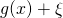whereis a standard Gaussian. Can you design a non-adaptive query strategy such that after at most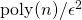(with possibly extra logarithmic factors) you will be able to correctly decide ifequalsor not with probability at least? In the paper we give a positive answer by constructing a mapwhereis a distribution supported on the domain ofwhich “exploresat every scale simultaneously”. At this point this map is still somewhat mysterious, and there are many open questions around it (can you compute the map efficiently? can this be useful beyond Bayesian convex bandits? can you find a better construction than ours? what is the optimal number of queries?).  See the paper for all the details!

This entry was posted in Optimization. Bookmark the permalink.

## 2 Responses to "A solution to bandit convex optimization"

••